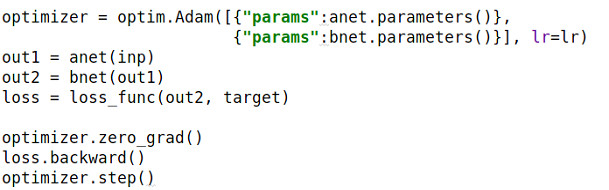# Backprop through weights of a second network

Hi, my question might be bit odd but i’m hoping it is possible with pytorch
Say i have two networks, the first of which outputs a bunch of values, one for each parameter of the second network (say a classification network) i then add these values to the parameters of the second network and perform a forward pass of the second network and compute a loss, is it possible to backprop the loss through to the first network to perform an optimization step?
Thanks

Yes, the first network can be optimized by the loss.

Ok, how would you do it?
I’m currently looping through the parameters of the second network and adding on the output of the first, but when I backprop the loss and step the optimizer (which has the parameters of the first network) the parameters of the first network don’t changeHope it helps you out.

Hi Ran,
unfortunately thats not quite what i’m after, i know you can backprop through the input of the second network, but what i’d like to do is to add the output of the first to the weights and bias of the second and then forward pass a separate input to the second network. see example below

``````def add_weights(weights, network):
c = 0
for p in network.parameters():
num_weights = p.data.view(-1).shape
params = weights[c:c+num_weights].view(p.data.shape)
c +=num_weights
p.data += params
return network

def SR_loss(network, images, labels, params):